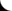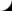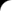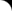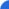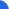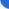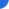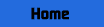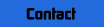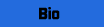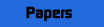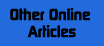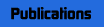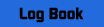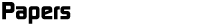Report On Phoenix Light Arrays

Page | 1 | 2 | 3 | 4 | 5

DURATIONS AND MOTION OF THE TRIANGLE LIGHTS

The lights in the triangle lasted between 4 min 20 sec and 4 min 45 sec. Analysis of changes in light position with time in the L video shows that they all dropped several thousand feet while drifting to the east at roughly 40 mph.

The relative locations of these preceding lights are illustrated in the FIGURE 11 which shows the linear array, the far right lights and the light triangle. All these light arrays were far from the witnesses, at high altitude, and basically over the northeast portion of the Air Force range, as can be seen by comparing with FIGURE 13, a map of the Phoenix area which is to the same scale as FIGURE 10. (To make this comparison you can use a light for transillumination and overlay FIGURE 10 on FIGURE 13 and align the obvious roads. Then observe the locations of the lights as compared to the boundaries of the Air Force test range.)

THE LIGHTS OF MARCH 13, 1997

The lights of 10:00 PM March 13, 1997 have been studied in a similar manner. In this case only the K, L and R videos have been used (P did not see the March 13 lights). FIGURES 14, 15, 16 and 17 show the light arrays. Two frames of the K video are shown because the second and third lights at the right side disappeared before the array was complete.

As before, the K and L sighting directions are quite accurate because of clear geographical reference points that appear in the videos. However, there is an ambiguity in determining exactly which lights are which in the two videos because L's videotape shows only three lights (another 3 had disappeared before L switched on the camera) whereas K' shows a total of 9 which appeared one after another in the distinctive array. An attempt at correlation by measuring the times between light extinctions was only slightly successful. Although one might assume that L videotaped the last three lights visible in the K or R videos, the fact is that there appears to be no convincing one-to-one correlation between specific lights in the K and L videos. However, if one merely assumes that L videotaped several of the lights in the K array one can determine the general location of the lights.

The R video shows an array that is almost identical to the K video array. However, as before, it is more ambiguous as to sighting directions because the reference points are indistinct and the angle calibration of the video screen depends upon an indistinct image (see discussion above). However, one can use the January 14, 1998 linear array lights (or the triangle) to remove some of the direction ambiguity. Specifically, the direction of the reference feature (roof of a house, see FIGURE 4) has been chosen (as above) in such a way as to make the sighting line to the left end light of the January 14 linear array pass through the location of the left end light as determined by the K and L videos (see FIGURES 9 and 10). (In other words, the January 14, 1998 sighting direction data have been used to calibrate the March 13, 1997 data.) The resulting data for lights numbered 1, 2 and 9 (in order of appearance) are shown below:

TABLE 6: MARCH 13, 1997 ARRAY OF LIGHTS as seen by K, L and R

 AZIMUTH CALCULATED (+/- 1 degree) DISTANCE (+/- 5 miles) FROM K FROM R FROM K FROM R left light 202 226 71 79 right end 207 229 75 85 between 1 and 2 205 226.7 77 86

The L video shows three lights which might be 7, 8, and 9, with sighting lines 210.5, 211 and 211.5 degrees. These lines pass through the area defined by the K and R videos.

The locations of the lights as determined from the above data are very roughly near, but farther away than, the location of the left hand ,linear array light discussed above (FIGURE 18). These data clearly point toward lights that are far from the observers and which are over the Air Force range.

The size of the array is given by the following estimated distances: between the first and second lights to appear was about 7.5 miles and between the second and ninth lights is about 3.7 miles (see FIGURE 18).

Page | 1 | 2 | 3 | 4 | 5

Top of Page